# NCERT Exemplar Class 12 Physics Solutions for Chapter 11 - Dual Nature of Radiation and Matter

NCERT Class 12 Physics Exemplar Solutions for Chapter 11 Dual Nature of Radiation and Matter provide explanations for the important topics. This exemplar solution, prepared by subject experts at BYJU’S, comprises Dual Nature of Radiation and Matter board questions, Dual Nature of Radiation and Matter important questions PDF and Dual Nature of Matter and Radiation entrance questions.

It is mandatory for students to solve these exemplar questions in order to grasp topics crucial from the exam point of view and score good marks in the CBSE Class 12 board exam and graduate entrance examinations.

## Download the PDF of the NCERT Exemplar Class 12 Physics Chapter 11 Dual Nature of Radiation and Matter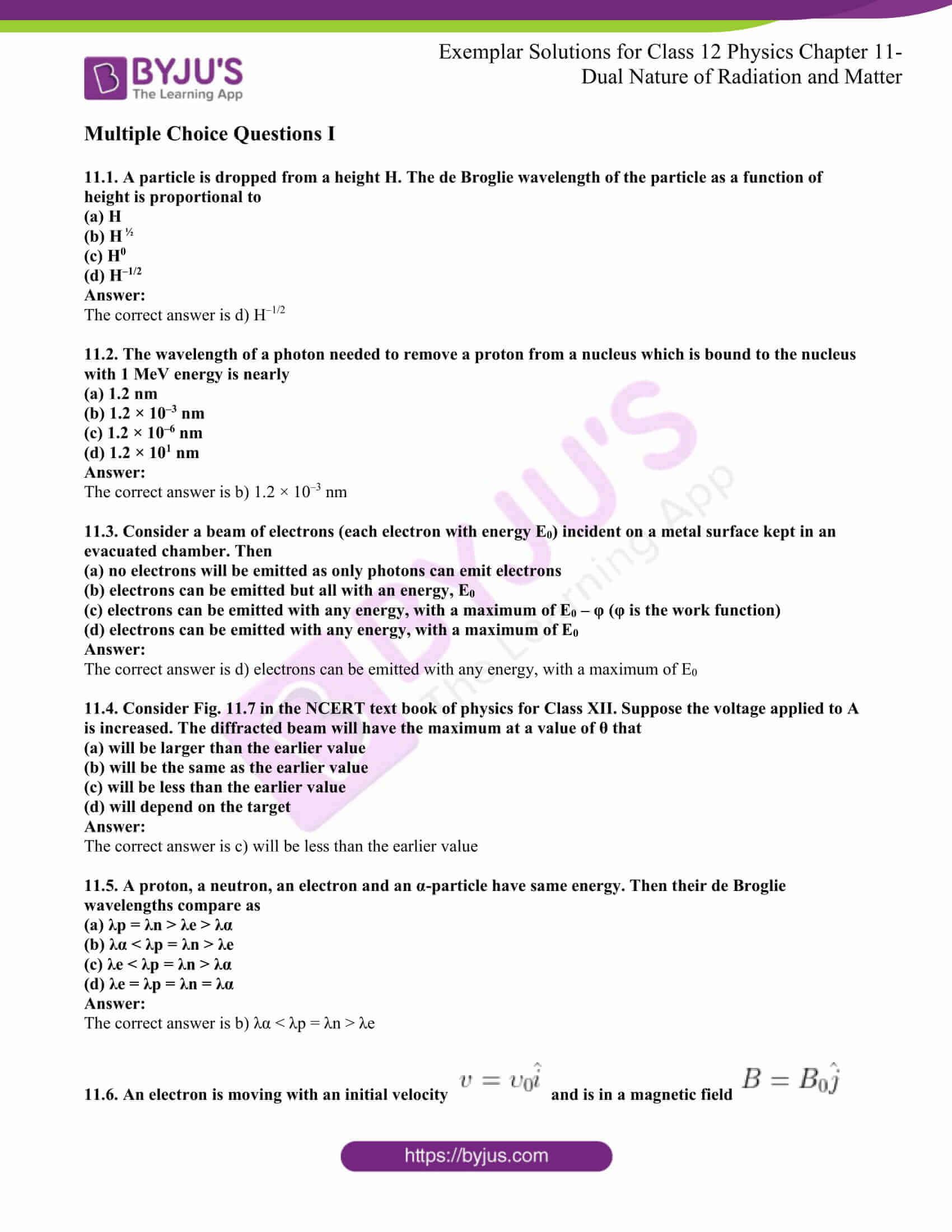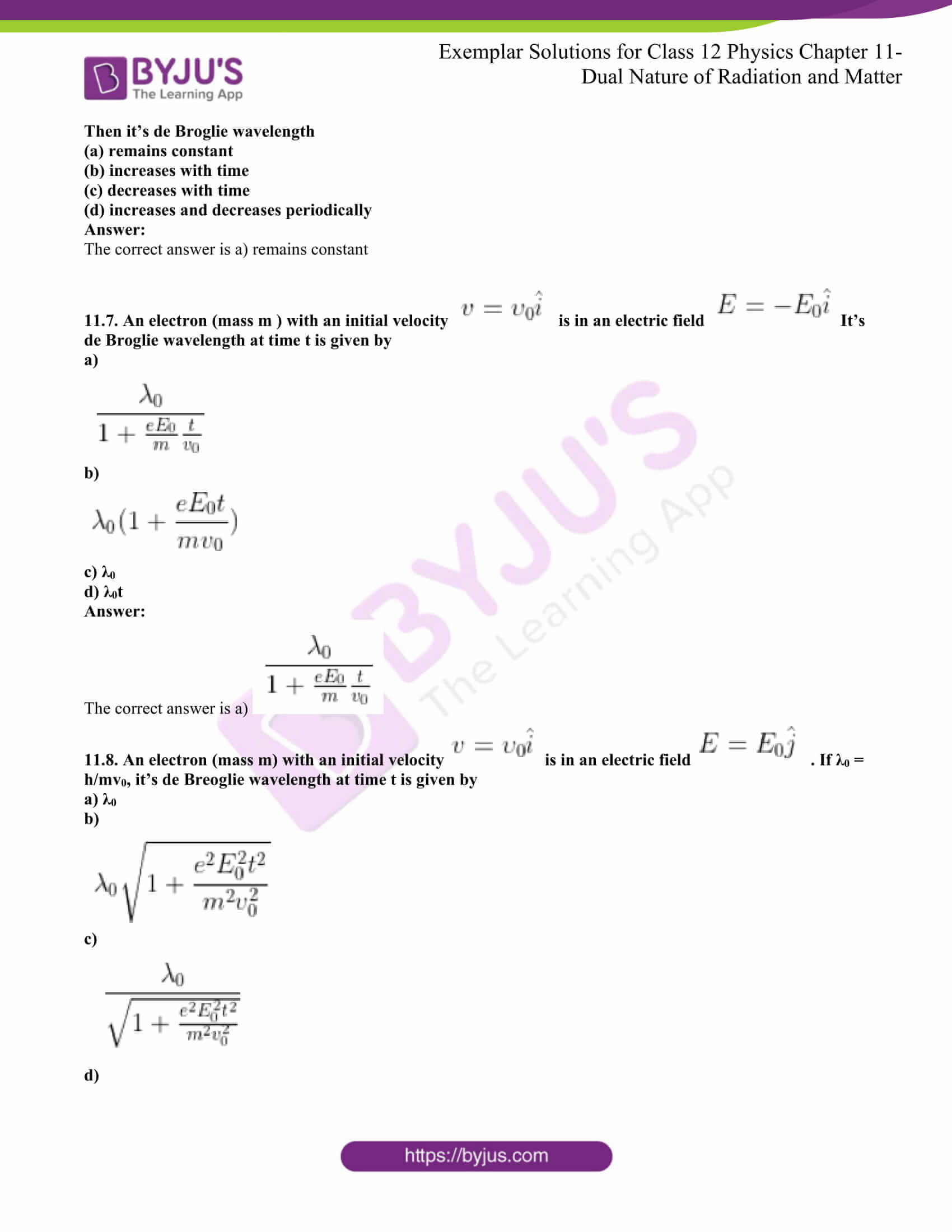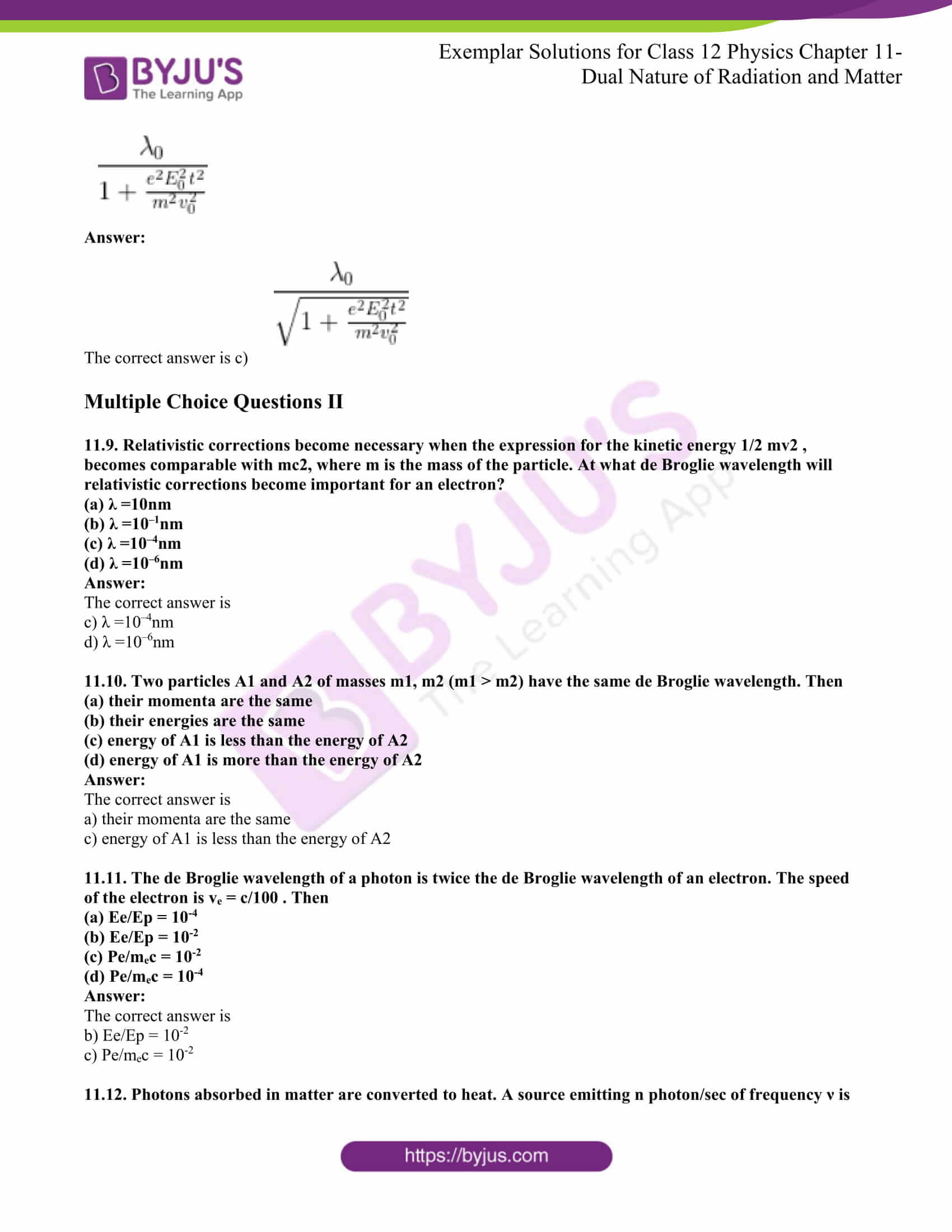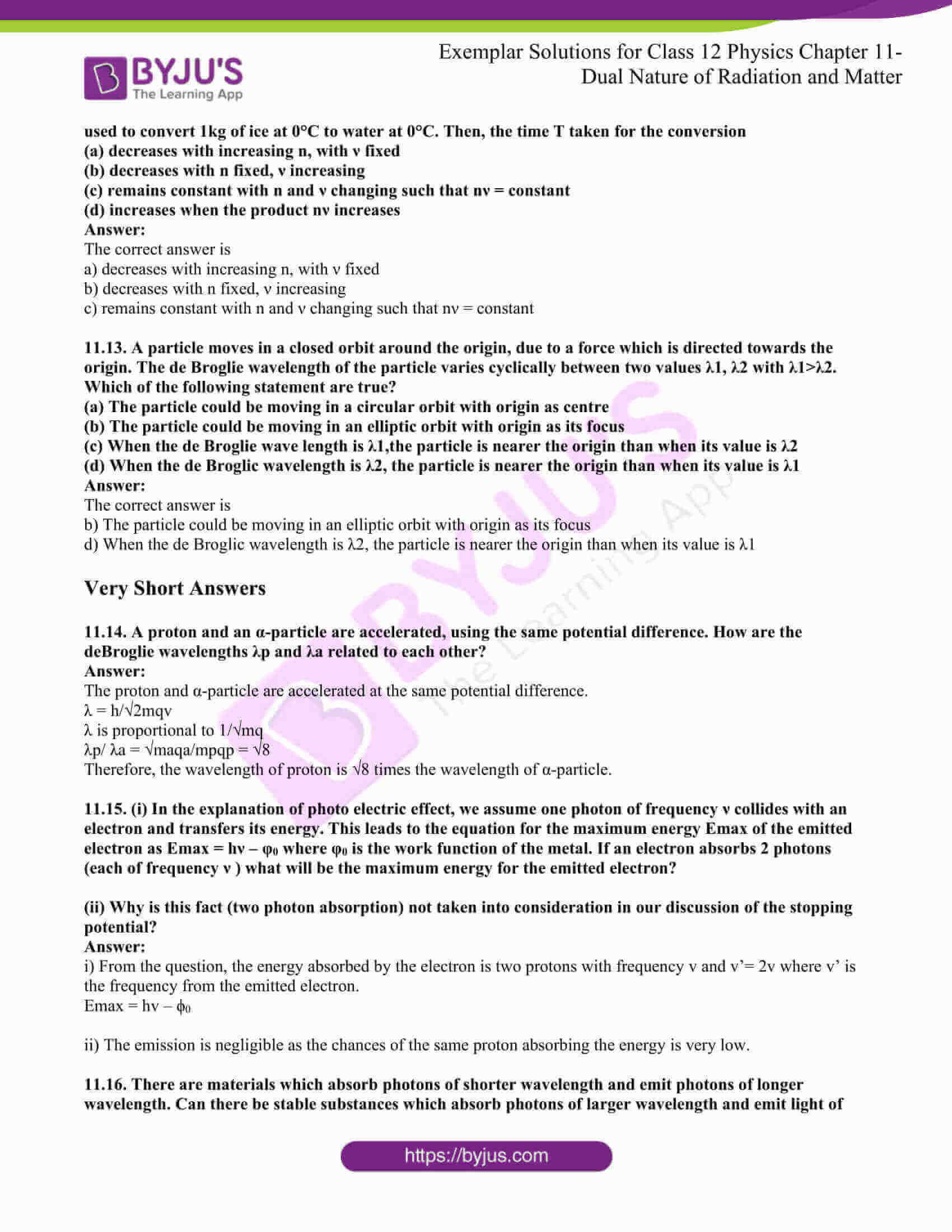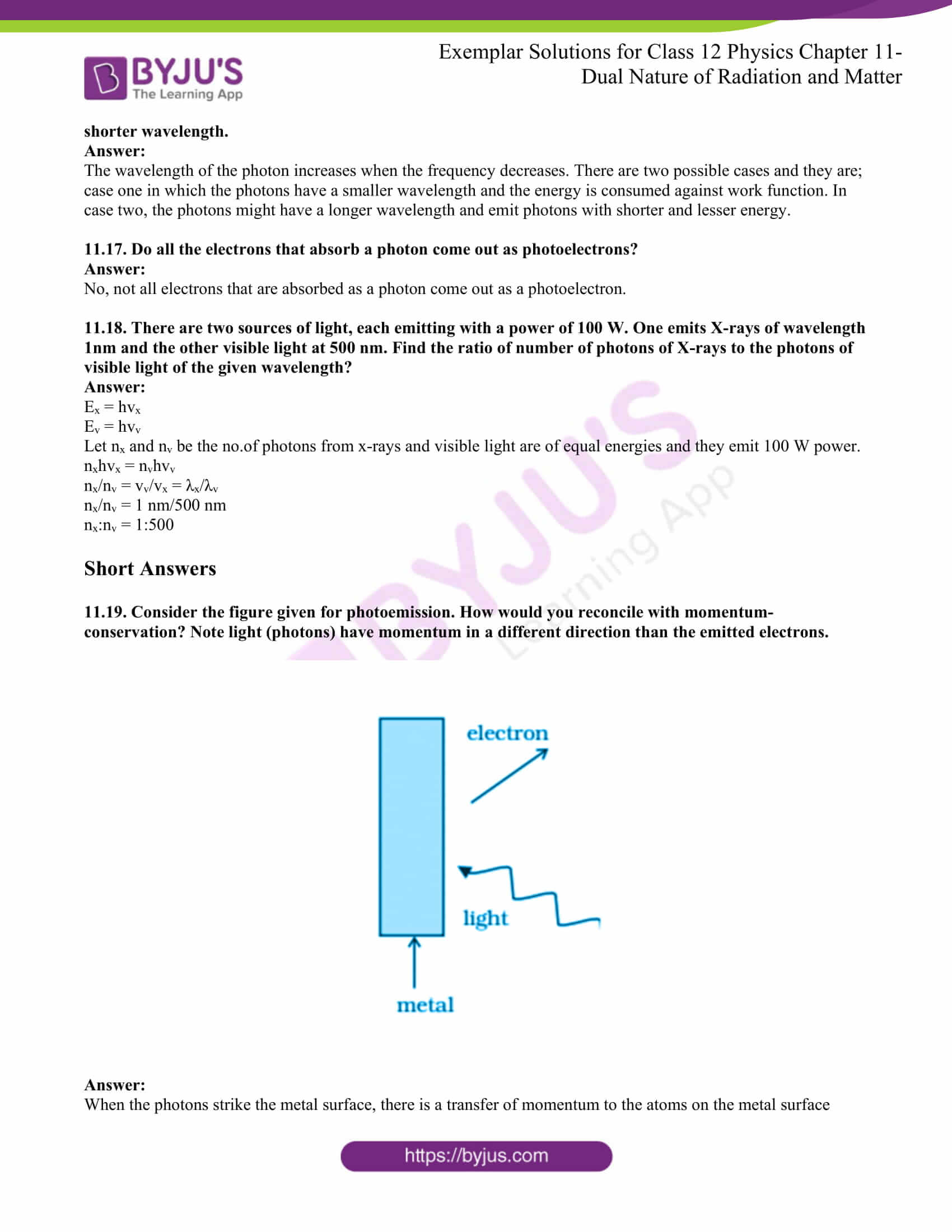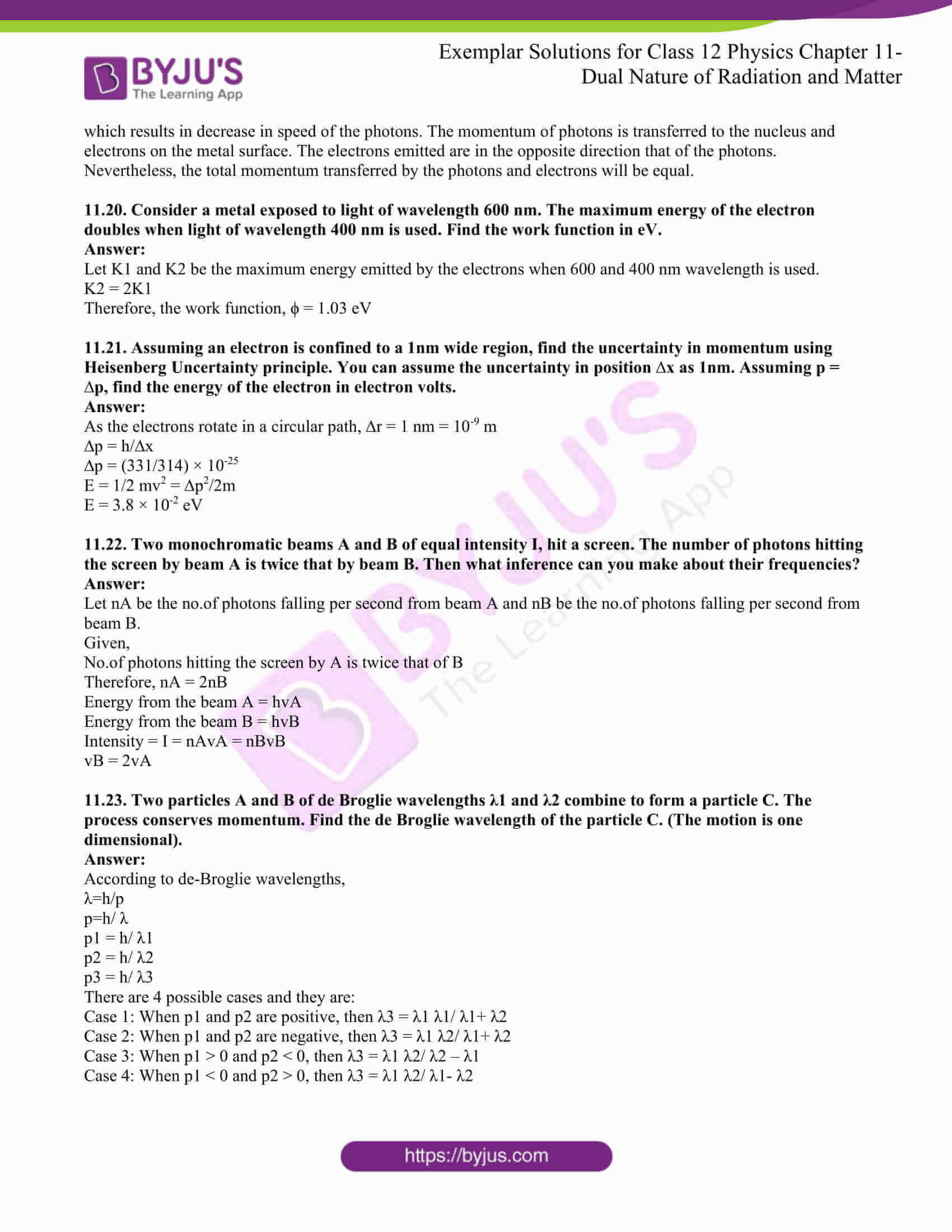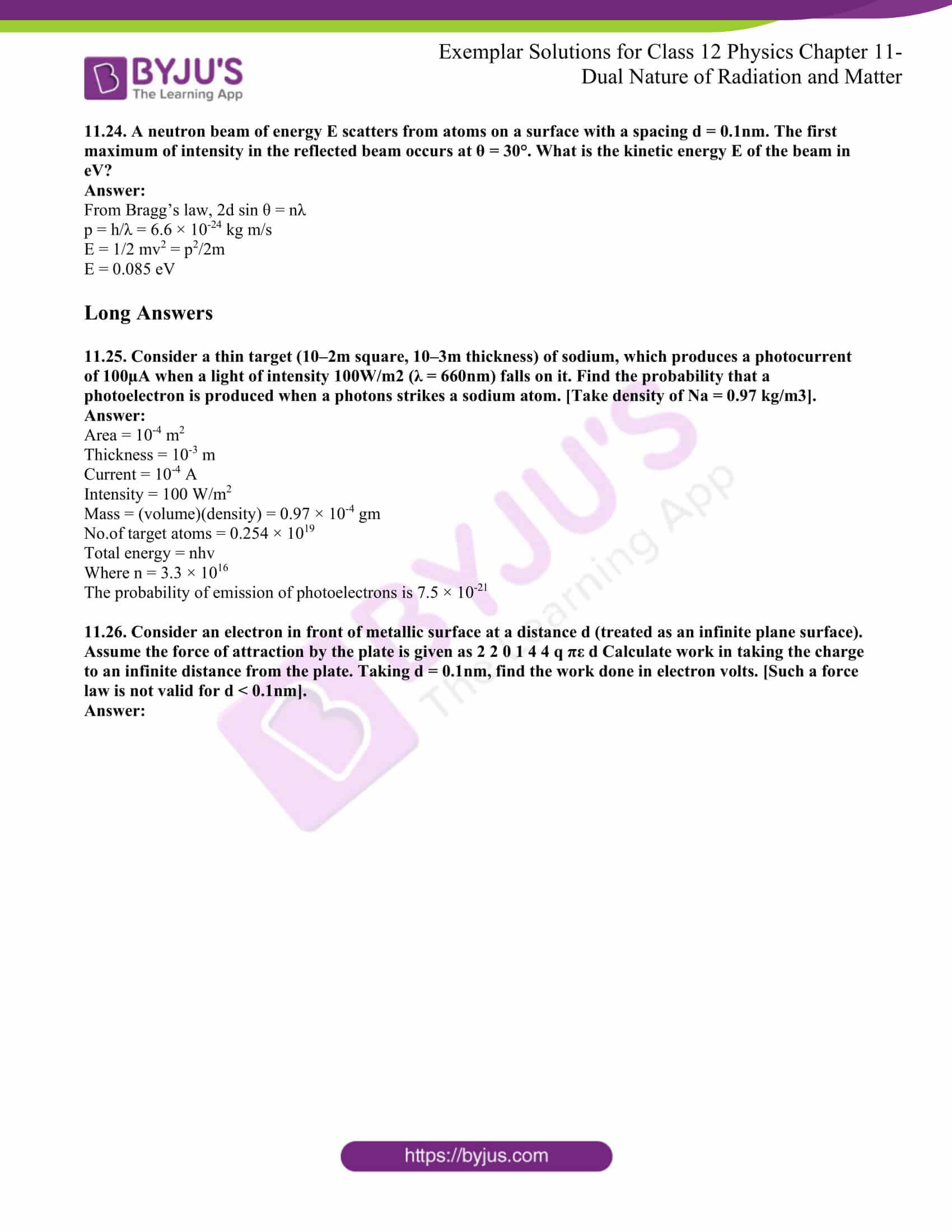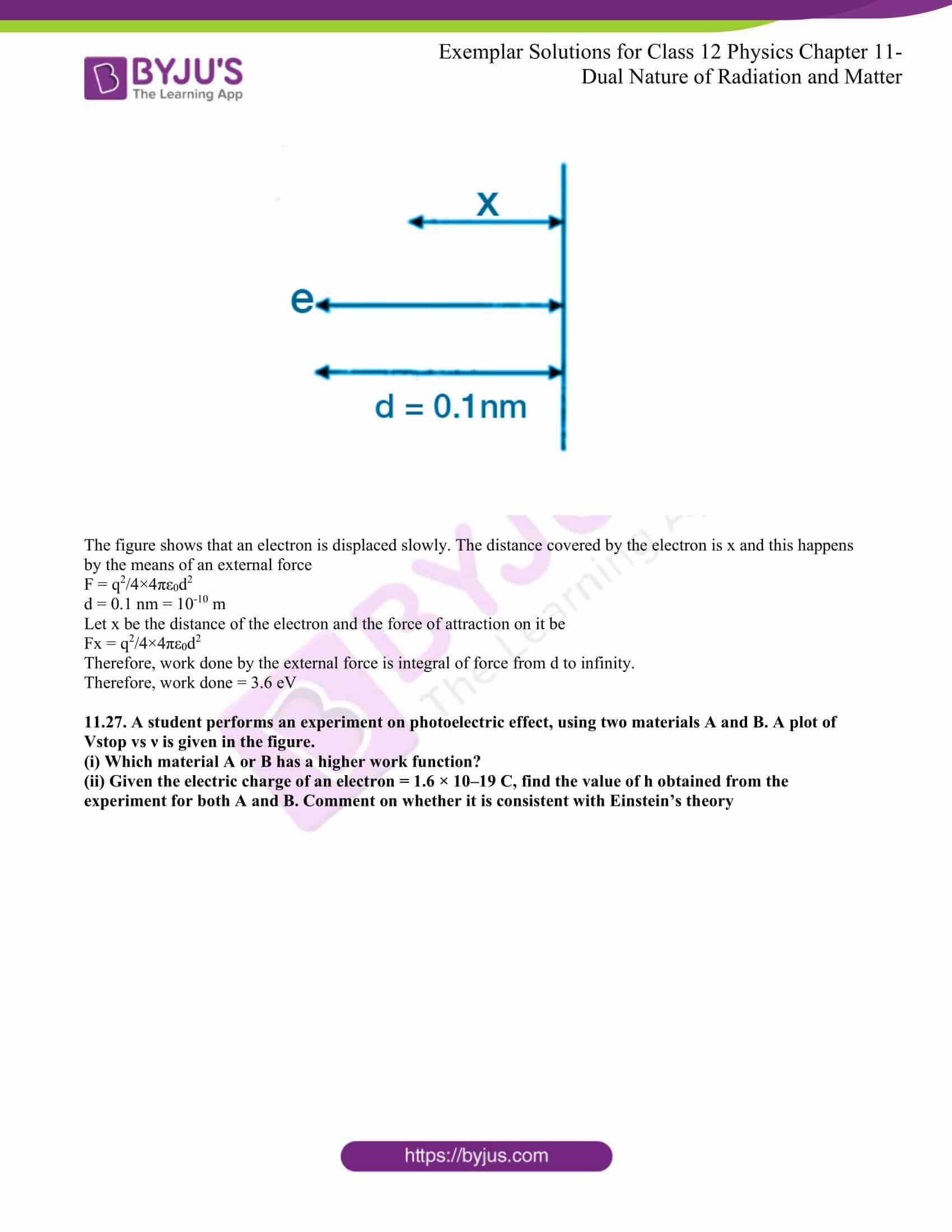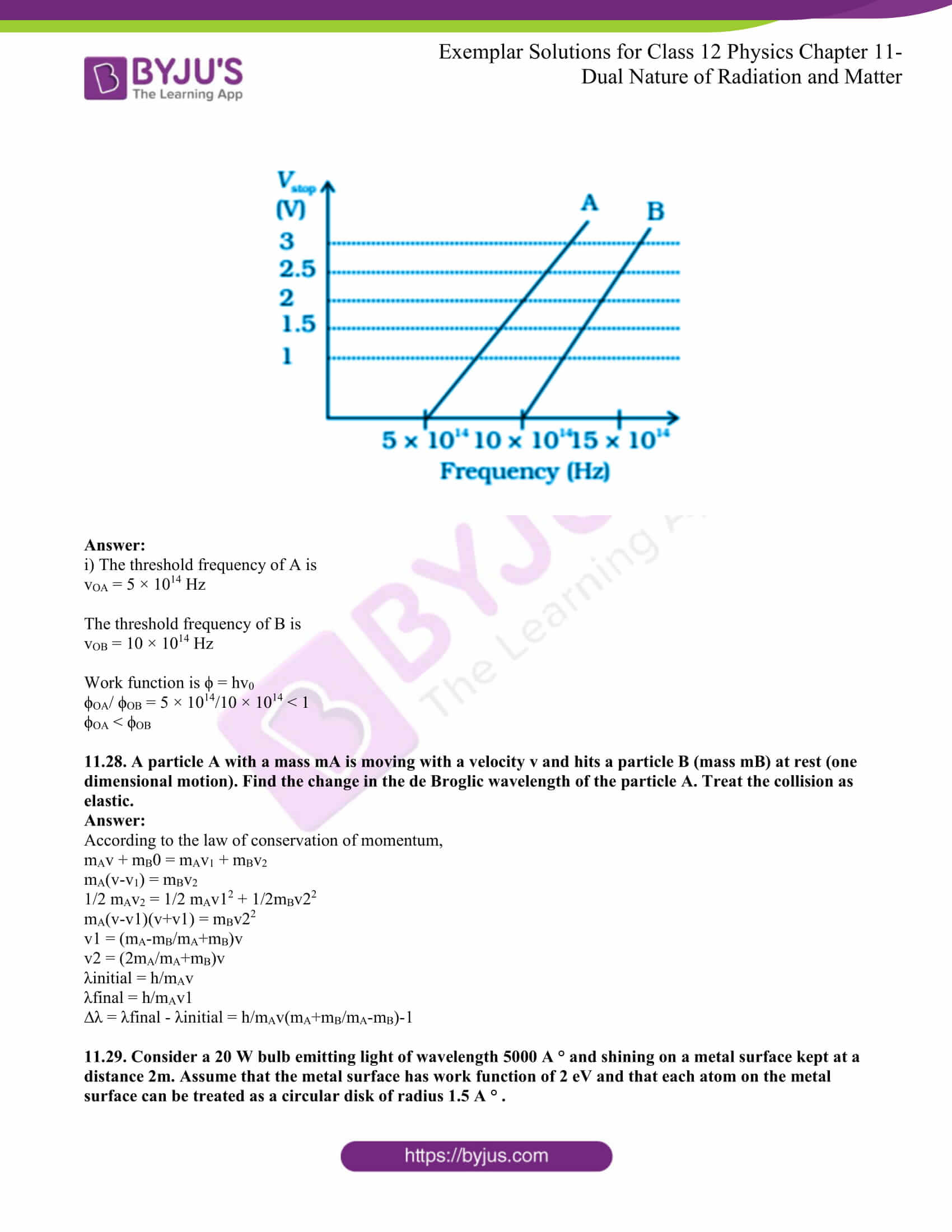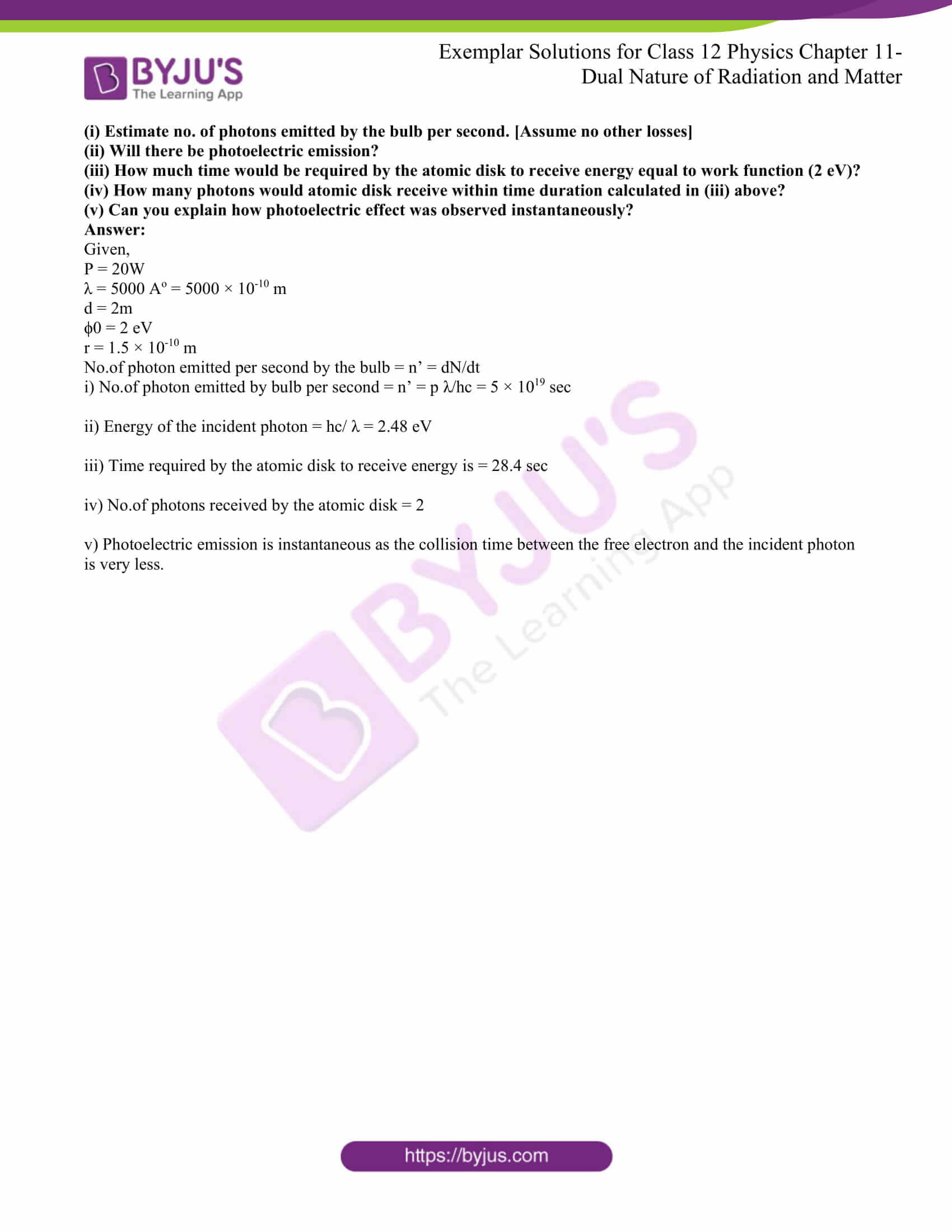### Multiple Choice Questions I

11.1. A particle is dropped from a height H. The de Broglie wavelength of the particle as a function of height is proportional to

(a) H

(b) H ½

(c) H0

(d) H–1/2

The correct answer is d) H–1/2

11.2. The wavelength of a photon needed to remove a proton from a nucleus which is bound to the nucleus with 1 MeV energy is nearly

(a) 1.2 nm

(b) 1.2 × 10–3 nm

(c) 1.2 × 10–6 nm

(d) 1.2 × 101 nm

The correct answer is b) 1.2 × 10–3 nm

11.3. Consider a beam of electrons (each electron with energy E0) incident on a metal surface kept in an evacuated chamber. Then

(a) no electrons will be emitted as only photons can emit electrons

(b) electrons can be emitted but all with an energy, E0

(c) electrons can be emitted with any energy, with a maximum of E0 – φ (φ is the work function)

(d) electrons can be emitted with any energy, with a maximum of E0

The correct answer is d) electrons can be emitted with any energy, with a maximum of E0

11.4. Consider Fig. 11.7 in the NCERT textbook of physics for Class XII. Suppose the voltage applied to A is increased. The diffracted beam will have the maximum at a value of θ that

(a) will be larger than the earlier value

(b) will be the same as the earlier value

(c) will be less than the earlier value

(d) will depend on the target

The correct answer is c) will be less than the earlier value

11.5. A proton, a neutron, an electron and an α-particle have the same energy. Then their de Broglie wavelengths compare as

(a) λp = λn > λe > λα

(b) λα < λp = λn < λe

(c) λe < λp = λn > λα

(d) λe = λp = λn = λα

The correct answer is b) λα < λp = λn < λe

11.6. An electron is moving with an initial velocity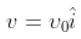and is in a magnetic field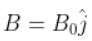Then it’s de Broglie wavelength

(a) remains constant

(b) increases with time

(c) decreases with time

(d) increases and decreases periodically

The correct answer is a) remains constant

11.7. An electron (mass m ) with an initial velocity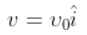is in an electric field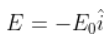It’s de Broglie wavelength at time t is given by

a)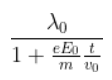b)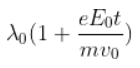c) λ0

d) λ0t

The correct answer is a)11.8. An electron (mass m) with an initial velocity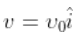is in an electric field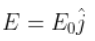. If λ0 = h/mv0, it’s de Broglie wavelength at time t is given by

a) λ0

b)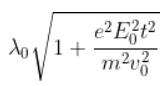c)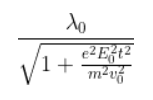d)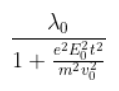The correct answer is c)### Multiple Choice Questions II

11.9. Relativistic corrections become necessary when the expression for the kinetic energy 1/2 mv2 , becomes comparable with mc2, where m is the mass of the particle. At what de Broglie wavelength will relativistic corrections become important for an electron?

(a) λ =10nm

(b) λ =10–1nm

(c) λ =10–4nm

(d) λ =10–6nm

c) λ =10–4nm

d) λ =10–6nm

11.10. Two particles A1 and A2 of masses m1, m2 (m1 > m2) have the same de Broglie wavelength. Then

(a) their momenta are the same

(b) their energies are the same

(c) energy of A1 is less than the energy of A2

(d) energy of A1 is more than the energy of A2

a) their momenta are the same

c) energy of A1 is less than the energy of A2

11.11. The de Broglie wavelength of a photon is twice the de Broglie wavelength of an electron. The speed of the electron is ve = c/100. Then

(a) Ee/Ep = 10-4

(b) Ee/Ep = 10-2

(c) Pe/mec = 10-2

(d) Pe/mec = 10-4

b) Ee/Ep = 10-2

c) Pe/mec = 10-2

11.12. Photons absorbed in matter are converted to heat. A source emitting n photon/sec of frequency ν is used to convert 1kg of ice at 0°C to water at 0°C. Then, the time T taken for the conversion

(a) decreases with increasing n, with ν fixed

(b) decreases with n fixed, ν increasing

(c) remains constant with n and ν changing such that nν = constant

(d) increases when the product nν increases

a) decreases with increasing n, with ν fixed

b) decreases with n fixed, ν increasing

c) remains constant with n and ν changing such that nν = constant

11.13. A particle moves in a closed orbit around the origin, due to a force which is directed towards the origin. The de Broglie wavelength of the particle varies cyclically between two values λ1, λ2 with λ1>λ2. Which of the following statement are true?

(a) The particle could be moving in a circular orbit with origin as centre

(b) The particle could be moving in an elliptic orbit with origin as its focus

(c) When the de Broglie wavelength is λ1, the particle is nearer the origin than when its value is λ2

(d) When the de Broglie wavelength is λ2, the particle is nearer the origin than when its value is λ1

b) The particle could be moving in an elliptic orbit with origin as its focus

d) When the de Broglie wavelength is λ2, the particle is nearer the origin than when its value is λ1

11.14. A proton and an α-particle are accelerated, using the same potential difference. How are the de Broglie wavelengths λp and λa related to each other?

The proton and α-particle are accelerated at the same potential difference.

λ = h/√2mqv

λ is proportional to 1/√mq

λp/ λa = √maqa/mpqp = √8

Therefore, the wavelength of the proton is √8 times the wavelength of α-particle.

11.15. (i) In the explanation of the photoelectric effect, we assume one photon of frequency ν collides with an electron and transfers its energy. This leads to the equation for the maximum energy Emax of the emitted electron as Emax = hν – φ0 where φ0 is the work function of the metal. If an electron absorbs 2 photons (each of frequency ν ) what will be the maximum energy for the emitted electron?

(ii) Why is this fact (two-photon absorption) not taken into consideration in our discussion of the stopping potential?

i) From the question, the energy absorbed by the electron is two protons with frequency v and v’= 2v where v’ is the frequency from the emitted electron.

Emax = hv – ϕ0

ii) The emission is negligible as the chances of the same proton absorbing the energy is very low.

11.16. There are materials which absorb photons of shorter wavelength and emit photons of longer wavelength. Can there be stable substances which absorb photons of larger wavelength and emit light of shorter wavelength?

The wavelength of the photon increases when the frequency decreases. There are two possible cases and they are; case one in which the photons have a smaller wavelength and the energy is consumed against work function. In case two, the photons might have a longer wavelength and emit photons with shorter and lesser energy.

11.17. Do all the electrons that absorb a photon come out as photoelectrons?

No, not all electrons that are absorbed as a photon come out as a photoelectron.

11.18. There are two sources of light, each emitting with a power of 100 W. One emits X-rays of wavelength 1nm and the other visible light at 500 nm. Find the ratio of a number of photons of X-rays to the photons of visible light of the given wavelength?

Ex = hvx

Ev = hvv

Let nx and nv be the no.of photons from x-rays and visible light are of equal energies and they emit 100 W power.

nxhvx = nvhvv

nx/nv = vv/vx = λxv

nx/nv = 1 nm/500 nm

nx:nv = 1:500

11.19. Consider the figure given for photoemission. How would you reconcile with momentum-conservation? Note light (photons) have momentum in a different direction than the emitted electrons.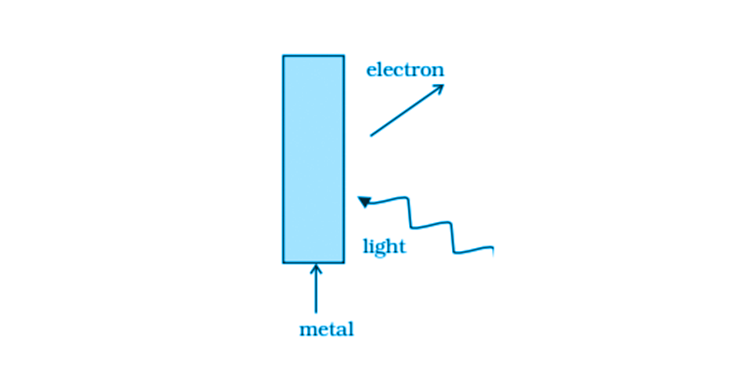When the photons strike the metal surface, there is a transfer of momentum to the atoms on the metal surface which results in a decrease in speed of the photons. The momentum of photons is transferred to the nucleus and electrons on the metal surface. The electrons emitted are in the opposite direction that of the photons. Nevertheless, the total momentum transferred by the photons and electrons will be equal.

11.20. Consider a metal exposed to light of wavelength 600 nm. The maximum energy of the electron doubles when light of wavelength 400 nm is used. Find the work function in eV.

Let K1 and K2 be the maximum energy emitted by the electrons when 600 and 400 nm wavelength is used.

K2 = 2K1

Therefore, the work function, ϕ = 1.03 eV

11.21. Assuming an electron is confined to a 1nm wide region, find the uncertainty in momentum using the Heisenberg Uncertainty principle. You can assume the uncertainty in position ∆x as 1nm. Assuming p = ∆p, find the energy of the electron in electron volts.

As the electrons rotate in a circular path, ∆r = 1 nm = 10-9 m

∆p = h/∆x

∆p = (331/314) × 10-25

E = 1/2 mv2 = ∆p2/2m

E = 3.8 × 10-2 eV

11.22. Two monochromatic beams A and B of equal intensity I, hit a screen. The number of photons hitting the screen by beam A is twice that by beam B. Then what inference can you make about their frequencies?

Let nA be the no.of photons falling per second from beam A and nB be the no.of photons falling per second from beam B.

Given,

No.of photons hitting the screen by A is twice that of B

Therefore, nA = 2nB

Energy from the beam A = hvA

Energy from the beam B = hvB

Intensity = I = nAvA = nBvB

vB = 2vA

11.23. Two particles A and B of de Broglie wavelengths λ1 and λ2 combine to form a particle C. The process conserves momentum. Find the de Broglie wavelength of the particle C. (The motion is one dimensional).

According to de-Broglie wavelengths,

λ=h/p

p=h/ λ

p1 = h/ λ1

p2 = h/ λ2

p3 = h/ λ3

There are 4 possible cases and they are:

Case 1: When p1 and p2 are positive, then λ3 = λ1 λ1/ λ1+ λ2

Case 2: When p1 and p2 are negative, then λ3 = λ1 λ2/ λ1+ λ2

Case 3: When p1 > 0 and p2 < 0, then λ3 = λ1 λ2/ λ2 – λ1

Case 4: When p1 < 0 and p2 > 0, then λ3 = λ1 λ2/ λ1- λ2

11.24. A neutron beam of energy E scatters from atoms on a surface with a spacing d = 0.1nm. The first maximum of intensity in the reflected beam occurs at θ = 30°. What is the kinetic energy E of the beam in eV?

From Bragg’s law, 2d sin θ = nλ

p = h/λ = 6.6 × 10-24 kg m/s

E = 1/2 mv2 = p2/2m

E = 0.085 eV

11.25. Consider a thin target (10–2m square, 10–3m thickness) of sodium, which produces a photocurrent of 100µA when a light of intensity 100W/m2 (λ = 660nm) falls on it. Find the probability that a photoelectron is produced when a photon strikes a sodium atom. [Take density of Na = 0.97 kg/m3].

Area = 10-4 m2

Thickness = 10-3 m

Current = 10-4 A

Intensity = 100 W/m2

Mass = (volume)(density) = 0.97 × 10-4 gm

No.of target atoms = 0.254 × 1019

Total energy = nhv

Where n = 3.3 × 1016

The probability of emission of photoelectrons is 7.5 × 10-21

11.26. Consider an electron in front of the metallic surface at a distance d (treated as an infinite plane surface). Assume the force of attraction by the plate is given as 2 2 0 1 4 4 q πε d Calculate work in taking the charge to an infinite distance from the plate. Taking d = 0.1nm, find the work done in electron volts. [Such a force law is not valid for d < 0.1nm].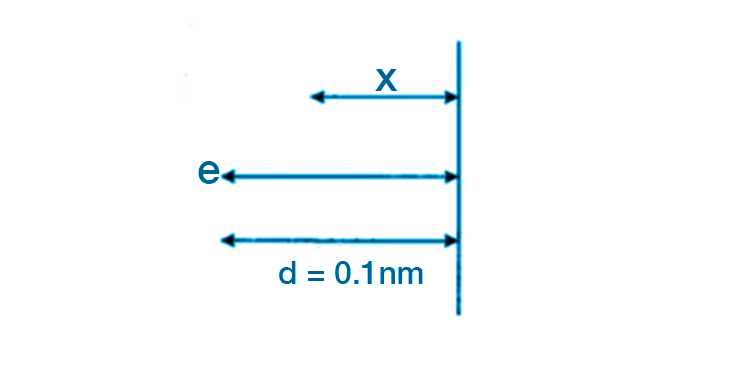The figure shows that an electron is displaced slowly. The distance covered by the electron is x and this happens by the means of an external force

F = q2/4×4πε0d2

d = 0.1 nm = 10-10 m

Let x be the distance of the electron and the force of attraction on it be

Fx = q2/4×4πε0d2

Therefore, work done by the external force is integral of force from d to infinity.

Therefore, work done = 3.6 eV

11.27. A student performs an experiment on the photoelectric effect, using two materials A and B. A plot of Vstop vs ν is given in the figure.

(i) Which material A or B has a higher work function?

(ii) Given the electric charge of an electron = 1.6 × 10–19 C, find the value of h obtained from the experiment for both A and B. Comment on whether it is consistent with Einstein’s theory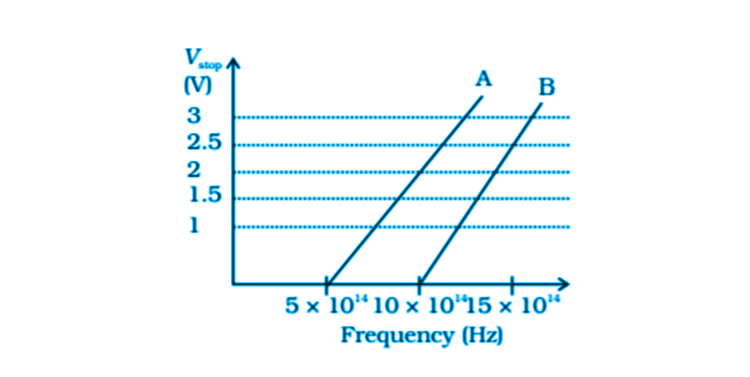i) The threshold frequency of A is

vOA = 5 × 1014 Hz

The threshold frequency of B is

vOB = 10 × 1014 Hz

Work function is ϕ = hv0

ϕOA/ ϕOB = 5 × 1014/10 × 1014 < 1

ϕOA < ϕOB

11.28. A particle A with a mass mA is moving with a velocity v and hits a particle B (mass mB) at rest (one dimensional motion). Find the change in the de Broglie wavelength of the particle A. Treat the collision as elastic.

According to the law of conservation of momentum,

mAv + mB0 = mAv1 + mBv2

mA(v-v1) = mBv2

1/2 mAv2 = 1/2 mAv12 + 1/2mBv22

mA(v-v1)(v+v1) = mBv22

v1 = (mA-mB/mA+mB)v

v2 = (2mA/mA+mB)v

λinitial = h/mAv

λfinal = h/mAv1

∆λ = λfinal – λinitial = h/mAv(mA+mB/mA-mB)-1

11.29. Consider a 20 W bulb emitting light of wavelength 5000 A ° and shining on a metal surface kept at a distance 2m. Assume that the metal surface has work function of 2 eV and that each atom on the metal surface can be treated as a circular disk of radius 1.5 A ° .

(i) Estimate no. of photons emitted by the bulb per second. [Assume no other losses]

(ii) Will there be photoelectric emission?

(iii) How much time would be required by the atomic disk to receive energy equal to work function (2 eV)?

(iv) How many photons would atomic disk receive within time duration calculated in (iii) above?

(v) Can you explain how photoelectric effect was observed instantaneously?

Given,

P = 20W

λ = 5000 Ao = 5000 × 10-10 m

d = 2m

ϕ0 = 2 eV

r = 1.5 × 10-10 m

No.of photon emitted per second by the bulb = n’ = dN/dt

i) No.of photon emitted by bulb per second = n’ = p λ/hc = 5 × 1019 sec

ii) Energy of the incident photon = hc/ λ = 2.48 eV

iii) Time required by the atomic disk to receive energy is = 28.4 sec

iv) No.of photons received by the atomic disk = 2

v) Photoelectric emission is instantaneous as the collision time between the free electron and the incident photon is very less.

The chapter Dual Nature of Radiation and Matter introduces students to the concept of matter that propagates through radiation. Under the close observation of a microscope, we can see that matter exists as both a wave, as well as a particle.

Some of the major topics covered in this chapter are

1. Einstein’s Photoelectric Emission
2. Emission of Electrons
3. Nature of Matter – Wave and Particle
4. Study of Photoelectric Effect through Experimentation
5. Davisson and Germer Experiment

Also Access

NCERT Solutions for Class 12 Maths Chapter 11

CBSE Notes for Class 12 Maths Chapter 11

### Why Opt for BYJU’S

BYJU’S is India’s best learning app that provides study materials, notes, assignments, worksheets, and video and animation lessons that help students memorise topics for a longer period of time. For all your queries on the CBSE Class 12 Physics topics and a great learning experience, visit BYJU’S website or download BYJU’S – The Learning App.